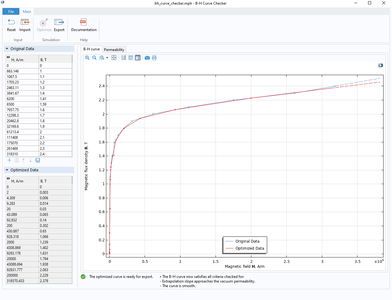# Application Gallery

## B-H Curve Checker

Application ID: 71651

This app demonstrates the following:

• Importing measured data from a text file
• Handling measured data using methods
• Exporting the results to a text file.

The app can be used to verify and optimize B-H curves using experimental data. It also generates curve data in the over-fluxed region, where measurement are difficult to perform. It removes the unphysical ripples of the slope of the B-H curve that might cause numerical instability.

The original B-H curve is evaluated from two aspects. Firstly, to verify that the extrapolation of the curve is reasonable from the physical point of view. Secondly, to check if the slope of the curve is smooth. The optimization algorithms are mainly based on the simultaneous exponential extrapolation method and the linear interpolation method, respectively.

The app requires the original curve data defined in a text input file. Once the curve is imported, the application checks if optimization is required. By clicking the 'Optimize Curve' button, the user can generate the optimized curve data, which can be exported to a text file.This application example illustrates applications of this type that would nominally be built using the following products: